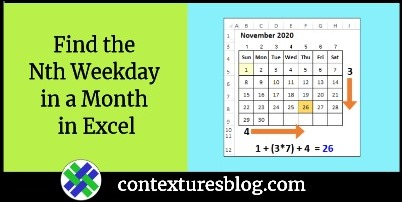## Find the Nth Weekday in a Month in Excel

Written by Debra Dalgleish from Contextures Blog

Some events occur on the Nth weekday of a specific month each year. Maybe your book club meets on the 4th Tuesday every month, and you don’t want to miss any meetings. In Excel, you can use a formula to find the Nth weekday in a month, and there’s an example shown below.

### Nth Weekday in a Month

Aside from your book club, there are other event that always occur on the Nth weekday in a month.

For example, here in Canada, we celebrate Victoria Day on the 3rd Monday in May, and in the USA. Thanksgiving is the 4th Thursday in November.

### Nth Weekday Formula

You can find the “Nth weekday in a month” dates in Excel, using a couple of Excel’s  date functions – WEEKDAY and DATE.

Here’s a screen shot of my sample file, with the named ranges that I created.Here’s the formula in cell C9, to calculate the 4th Thursday in November 2020. The formula uses the defined names, instead of cell references:

=DATE(SelYr,SelMth,1
+ ((SelN-(SelWD>= WEEKDAY(DATE(SelYr,SelMth,1)))) *7)
+ (SelWD- WEEKDAY(DATE(SelYr,SelMth,1))))

NOTE: That formula is based on the late Chip Pearson’s nth day example. Chip’s site shows the formula, but doesn’t explain how it works, so I made a page on my Contextures site, with details.

### How It Works

First, the Nth weekday formula calculates the 1st of the month date, with the DATE function.

• DATE(SelYr,SelMth,1)

It also calculates the weekday number for that month start date, using the WEEKDAY function:

• WEEKDAY(DATE(SelYr,SelMth,1)

### Find the Nth Occurrence

Next, the formula checks if the 1st occurrence is in the month’s 1st week, by comparing the 1st’s weekday number, to the target weekday number.

• If the 1st occurrence is in the 1st week, the formula adds (N-1) * 7 days to the start date
• If the 1st occurrence is NOT in the 1st week, the formula adds (N-0) * 7 days to the start date

In November 2020, the 1st Thursday is in the 1st week, so 21 days are added, to get to the week with the Nth occurrence:

• (4-1) * 7 = 21

### Find the Weekday

Next, the formula adds or subtracts days, based on the weekday numbers.

• If the target weekday is less than the month start weekday, days are subtracted
• If the target weekday is the same as the month start weekday, zero days are added
• If the target weekday is greater than the month start weekday, days are added

In November  2020, the 1st is on a Sunday (weekday 1) and the target day is a Thursday (weekday 5). The formula adds 4 days:

• 5 – 1 = 4

### Nth Weekday Formula Result

For November 2020, the 4th Thursday is on the 26th. Here’s the formula, with the numbers used to calculate that date:

• 1 + ((4-1) * 7) + 4 = 26### Get the Sample File

For more details on the formula, and a sample file, go to the Nth Weekday page on my Contextures site.

____________________

### Find the Nth Weekday in a Month in Excel____________________

Find the Nth Weekday in a Month in Excel is a post from Contextures Blog and is not allowed to be copied to other sites